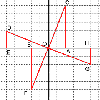# Search by Topic

#### Resources tagged with Matrices similar to Integration Matcher:

Filter by: Content type:
Age range:
Challenge level:

##### Other tags that relate to Integration Matcher
Integration. Biology. Vectors. Differentiation. Physics. Investigations. Matrices. Maths Supporting SET. Mathematical modelling. Curve Fitting.

### There are 14 results

Broad Topics > Vectors and Matrices > Matrices### Transformations for 10

##### Age 16 to 18 Challenge Level:

Explore the properties of matrix transformations with these 10 stimulating questions.### Fix Me or Crush Me

##### Age 16 to 18 Challenge Level:

Can you make matrices which will fix one lucky vector and crush another to zero?### Square Pair

##### Age 16 to 18 Challenge Level:

Explore the shape of a square after it is transformed by the action of a matrix.### Matrix Meaning

##### Age 16 to 18 Challenge Level:

Explore the meaning behind the algebra and geometry of matrices with these 10 individual problems.### Nine Eigen

##### Age 16 to 18 Challenge Level:

Explore how matrices can fix vectors and vector directions.### Stringing it Out

##### Age 14 to 16 Challenge Level:

Explore the transformations and comment on what you find.### Two and Four Dimensional Numbers

##### Age 16 to 18 Challenge Level:

Investigate matrix models for complex numbers and quaternions.### Rots and Refs

##### Age 16 to 18 Challenge Level:

Follow hints using a little coordinate geometry, plane geometry and trig to see how matrices are used to work on transformations of the plane.### Reflect Again

##### Age 16 to 18 Challenge Level:

Follow hints to investigate the matrix which gives a reflection of the plane in the line y=tanx. Show that the combination of two reflections in intersecting lines is a rotation.### 8 Methods for Three by One

##### Age 14 to 18 Challenge Level:

This problem in geometry has been solved in no less than EIGHT ways by a pair of students. How would you solve it? How many of their solutions can you follow? How are they the same or different?. . . .### The Matrix

##### Age 16 to 18 Challenge Level:

Investigate the transfomations of the plane given by the 2 by 2 matrices with entries taking all combinations of values 0. -1 and +1.### Matrix Countdown

##### Age 16 to 18 Challenge Level:

Play countdown with matrices### Limiting Probabilities

##### Age 16 to 18 Challenge Level:

Given probabilities of taking paths in a graph from each node, use matrix multiplication to find the probability of going from one vertex to another in 2 stages, or 3, or 4 or even 100.### The Golden Ratio, Fibonacci Numbers and Continued Fractions.

##### Age 14 to 16

An iterative method for finding the value of the Golden Ratio with explanations of how this involves the ratios of Fibonacci numbers and continued fractions.